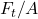The brake pads for a bicycle tire are made of rubber. If a frictional force of 50 N is applied to each side of the tires, determine the aver

Question

The brake pads for a bicycle tire are made of rubber. If a frictional force of 50 N is applied to each side of the tires, determine the average shear strain in the rubber. Each pad has cross-sectional dimensions of 20 mm and 50 mm. Gr

in progress 0
5 months 2021-08-25T17:14:57+00:00 1 Answers 209 views 0

average shear strain in the rubber is 0.25rad

Explanation:

The base has a dimension of 20mm and 50mm.

frictional force is 50 N

using the expressed shear stress,

we will determine τ =τ  = 50 / 1000

= 0.05MPa

shear stress  τ = γ .G

τ  = shear stress

γ  = shear strain

G  = 0.20MPa

γ = τ / G

= 0.05 / 0.20